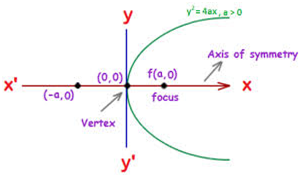×#### Thank you for registering.

One of our academic counsellors will contact you within 1 working day.

Click to Chat

1800-1023-196

+91-120-4616500

CART 0

• 0

MY CART (5)

Use Coupon: CART20 and get 20% off on all online Study Material

ITEM
DETAILS
MRP
DISCOUNT
FINAL PRICE
Total Price: Rs.

There are no items in this cart.
Continue Shopping• Complete JEE Main/Advanced Course and Test Series
• OFFERED PRICE: Rs. 15,900
• View Details

```Revision Notes on Parabola

The general equation of a conic is ax2 + 2hxy + by2 + 2gx + 2fy +c =0. Here if e =1 and D≠ 0, then it represents a parabola.

The general equation of parabola is (y-y0)2 = (x-x0), which has its vertex at (x0, y0).

The general equation of parabola with vertex at (0, 0) is given by y2 = 4ax, and it opens rightwards.

The parabolax2 = 4ay opens upwards.

The equation y2 = 4ax is considered to be the standard equation of the parabola for which the various components areVertex at (0,0)

Directrix is x+a = 0

Axis is y = 0

Focus is (a, 0)

Length of latus rectum = 4a

Ends of latus rectum are L(a, 2a) and L’(a, -2a)

The parabola y = a(x – h)2 + khas its vertex at (h, k)

The perpendicular distance from focus on directrix is half the length of latus rectum

Vertex is the middle point of the focus and the point of intersection of directrix and axis

Two parabolas are said to be equal if they have the same latus rectum

The point (x1, y1) lies outside, on or inside the parabolay2 = 4ax, according as the expression y12 = 4ax1 is positive, zero or negative.

Length of the chord intercepted by the parabola on the line y = mx + c is (4/m2) √a(1+m2) (a-mc)

Length of the focal chord which makes an angle δ with the x-axis is 4a cosec2δ

In parametric form, the parabola is represented by the equations x = at2 and y =2at

The equation of a chord joining t1 and t2 is given by 2x – (t1 + t2) y + 2at1t2 = 0

If a chord joining t1, t2 and t3, t4 pass through a point (c, 0) on the axis, then t1t2 = t3t4 = -c/a

Tangents to the parabola y2 = 4ax

yy1 = 2a(x+x1) at the point (x1, y1)

y = mx + a/m ( m ≠ 0) at (a/m2, 2a/m)

ty = x+at2 at (at2, 2at)

Normals to the parabola y2 = 4ax

y-y1 = -y1/ 2a(x-x1) at the point (x1, y1)

y = mx -2am – am3 at (am2, -2am)

y + tx = 2at +at3 at (at2, 2at)

The equation of the director circle to the parabola is x + a = 0 which is same as the equation of the directrix

The circle circumscribing the triangle formed by any three tangents to a parabola passes through the focus.

The orthocenter of any triangle formed by three tangents to a parabola y2 = 4ax lies on then directrix and has the coordinates –a, a(t1 + t2 + t3 + t1t2t3).

The area of the triangle formed by three points on a parabola is twice the area of the triangle formed by the tangents at these points.

A circle circumscribing the triangle formed by three co-normal points passes through the vertex of the parabola and its equation is given by

2(x2 + y2)– 2(h+2a)x - ky =0

The two vital parabolas along with their basic components like vertex and directrix are tabulated below:```### Course Features

• 728 Video Lectures
• Revision Notes
• Previous Year Papers
• Mind Map
• Study Planner
• NCERT Solutions
• Discussion Forum
• Test paper with Video Solution